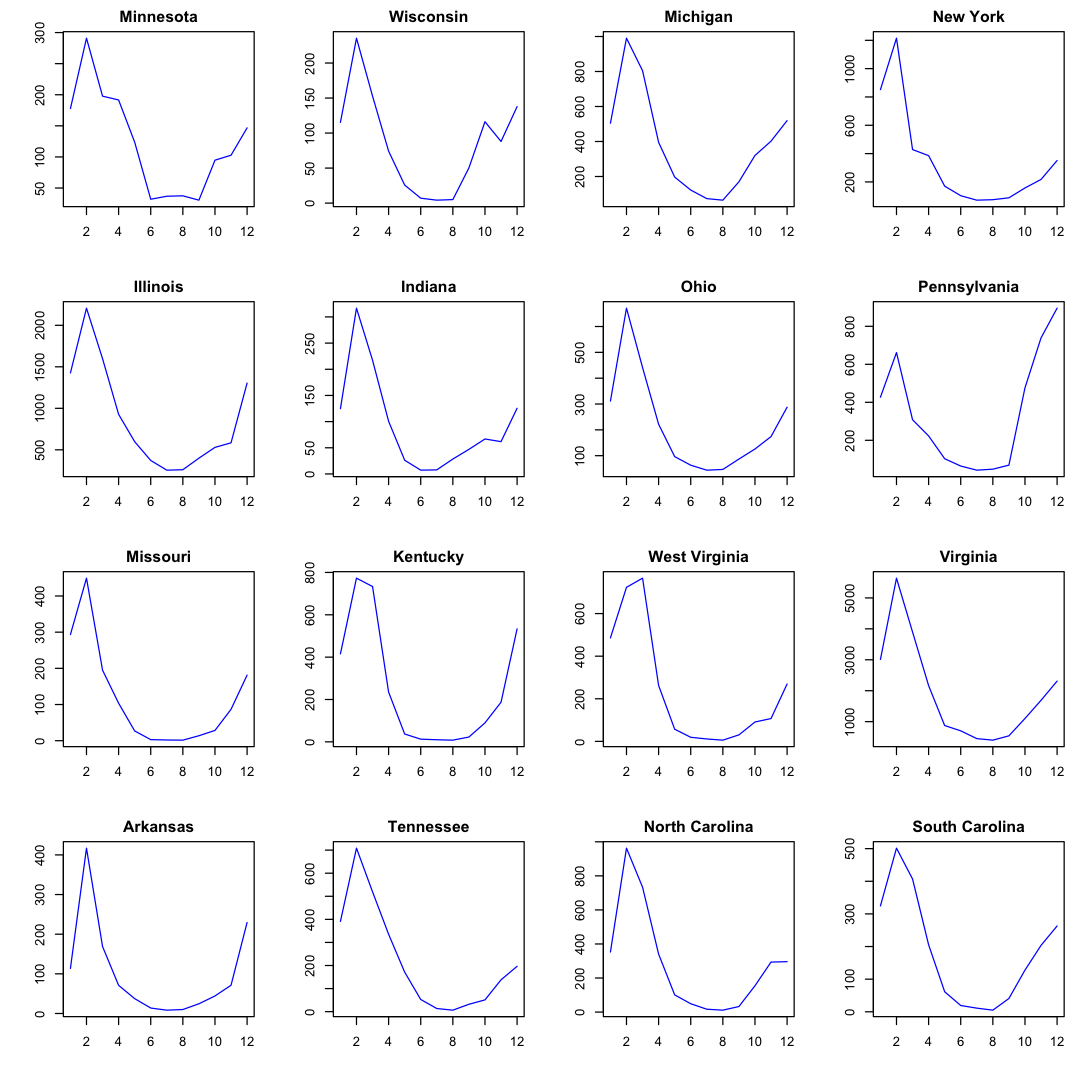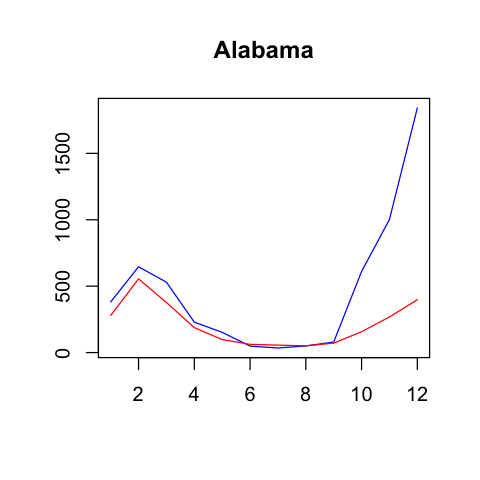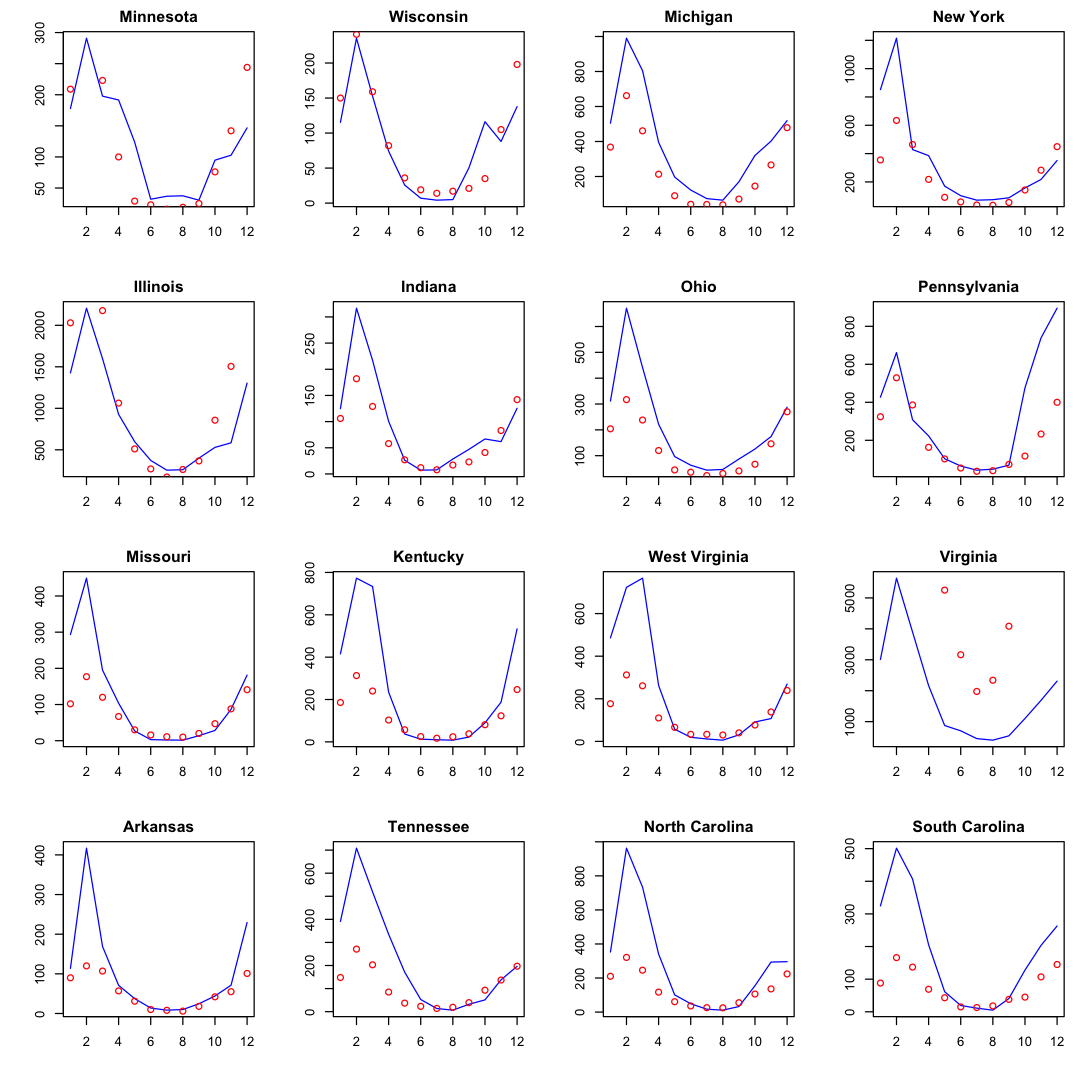# Flu Forecasting using GLM¶

Dec. 14, 2018 $\newcommand{\bs}{\boldsymbol}$ $\newcommand{\argmin}{\underset{\bs{#1}}{\text{arg min}}\,}$ $\newcommand{\argmax}{\underset{\bs{#1}}{\text{arg max}}\,}$ $\newcommand{\tr}{^{\top}}$ $\newcommand{\norm}{\left|\left|\,#1\,\right|\right|}$ $\newcommand{\given}{\,|\,}$ $\DeclareMathOperator{\E}{\mathbb{E}}$ $\newcommand{\blue}{{\color{blue} {#1}}}$ $\newcommand{\red}{{\color{red} {#1}}}$ $\newcommand{\orange}{{\color{orange} {#1}}}$ $\newcommand{\pfrac}{\frac{\partial #1}{\partial #2}}$

Let's apply our Poisson linear mixed model to a real world dataset. The dataset is downloaded from Center for Disease Control and Prevention (CDC)'s website. The source code from the previous article is loaded in a R file, and read below.

In :
source('GLM_Poisson.R')
library(tidyverse) # a set of packages that make R awesome, better than Python :D


## Data Preprocessing¶

In :
flu <- read_csv('fludata.csv', col_types = cols())
flu <- flu[flu['TEMPERATURE']!='X' & flu['ILITOTAL']!='X',] # get rid of missing values
flu[2:7] <- lapply(flu[2:7], as.numeric)

REGIONYEARWEEKTEMPERATUREILITOTALNUM. OF PROVIDERSTOTAL PATIENTS
Alabama 2010 40 64.3 249 35 11664
Arizona 2010 40 62.7 172 49 25492
Arkansas 2010 40 62.7 18 15 2586
California 2010 40 60.5 632 112 32342
Colorado 2010 40 49.2 134 14 20282
Connecticut2010 40 52.8 3 12 3831

For this project, we will focus on year 2017, using temperature and month as predictors. To achieve that, we divide week number by 4.33 to obtain an approximate month value for each data point. Then we use the average value of the predictors and response variables in each month based on the original data frame using the group_by method. The whole process can be achieved using the incredible dplyr package from the tidyverse. This is makes R the best language for manipulating small data frames. After hearing about tidyverse, I become deeply in love with R!

In :
df <- flu %>%
filter(YEAR==2017) %>%
mutate(MONTH=floor(WEEK/4.33)+1) %>%
filter(MONTH!=13) %>%
group_by(REGION, MONTH) %>%
summarise(COUNT=mean(ILITOTAL), TEMP=mean(TEMPERATURE), X0=1)


REGIONMONTHCOUNTTEMPX0
Alabama1 381.00 52.40 1
Alabama2 646.00 55.60 1
Alabama3 530.00 58.30 1
Alabama4 229.20 65.42 1
Alabama5 152.25 70.00 1
Alabama6 48.75 75.80 1
In :
# Plotting each group separately
options(repr.plot.width=9, repr.plot.height=9)
par(mfrow=c(4,4), mar=c(4, 4, 2, 1))
states = c('Minnesota', 'Wisconsin', 'Michigan', 'New York',
'Illinois', 'Indiana', 'Ohio', 'Pennsylvania',
'Missouri', 'Kentucky', 'West Virginia', 'Virginia',
'Arkansas', 'Tennessee', 'North Carolina', 'South Carolina'
)

for (i in 1:16){
df.state <- filter(df, REGION==states[i])
plot(df.state$COUNT, type='l', main=states[i], ylab='', xlab='', col='blue') }To fit our previously built model, we first need our data matrix in the form of $$\bs{X} = (\bs{X}_1\tr, \bs{X}_2\tr,..., \bs{X}_n\tr)'$$ where$\bs{X}_i$is the design matrix for the$i$th subject: $$\bs{X}_i = (\bs{x}_{i1},\bs{x}_{i2},...,\bs{x}_{i,m_i})'$$ This is automatically satisfied from the group_by method. We also need to load the design matrix$\bs{Z}$define by $$\bs{Z}=\text{diag}(\bs{1}_{m_1},..., \bs{1}_{m_n}),$$ where$n$is the number of states, and$m_i$is the number of training examples in state$i$. In : paste('There are', length(unique(df$REGION)), 'states.')%>%print() # number of states

 "There are 47 states."

In :
(group_by(df, REGION) %>% summarise(n = n()) %>% t())[,1:5]

 REGION n Alabama Arizona Arkansas California Colorado 12 12 12 12 12

Hence, we see that there are 47 states in the dataset, and each containing 12 data points. So we have $i=1,...,47$, and $j=1,...,12$ for each $i$. One more thing. If we directly use month numbers as the values of the predictor, then we will run into problems. This is because even though December (12) is higher than January (1) in value, this difference is meaningless in our situation. Instead, we need to use one-hot encoding.

In :
one_hot <- function(vector) {
m <- unique(vector) %>% length()
n <- length(vector)
I <- diag(m)
Z <- matrix(0, n, m)
for (i in 1:n) {
Z[i,] <- I[vector[i],] %>% as.vector()
}
as.tibble(Z) # data structure for tidyverse
}

In :
flu <- df %>%
bind_cols(one_hot(df$MONTH)) %>% select(REGION, X0, TEMP, 6:17, COUNT) head(flu)  REGIONX0TEMPV1V2V3V4V5V6V7V8V9V10V11V12COUNT Alabama1 52.40 1 0 0 0 0 0 0 0 0 0 0 0 381.00 Alabama1 55.60 0 1 0 0 0 0 0 0 0 0 0 0 646.00 Alabama1 58.30 0 0 1 0 0 0 0 0 0 0 0 0 530.00 Alabama1 65.42 0 0 0 1 0 0 0 0 0 0 0 0 229.20 Alabama1 70.00 0 0 0 0 1 0 0 0 0 0 0 0 152.25 Alabama1 75.80 0 0 0 0 0 1 0 0 0 0 0 0 48.75 ## Model Fitting¶ Now we are ready to initialize our model. The fixed effect$\bs{\theta}$now has dimension$12+2 = 14$. In : # initializing the model theta <- rep(0,14) # fixed effect vdiag <- list() for (i in 1:47){vdiag[[i]] <- rep(1,12)} Z <- bdiag(vdiag) %>% as.matrix() # building matrix Z X <- as.matrix(flu[2:15]) # design matrix y <- as.vector(flu[]) # response vector B <- rep(0,47) # subject random effects beta_i Gamma <- rep(0,47*12) # unit random effects gamma_ij sig1 <- 1; sig2 <- 10 # precision parameter a <- rep(0,14); R <- 10*diag(14) # prior for fixed effect  In : current.time <- proc.time() result <- Poisson.mcmc(theta, X, y, Z, B, Gamma, sig1, sig2, a, R, N=5000, thin=10, burnin=1000, seed=1, plot=TRUE) print("TOTAL RUNTIME") print(proc.time()-current.time)   "SUMMARY"  -------------------------  "Iterations: 5000"  "Burn-in: 1000"  "Thin: 10"  "Acceptance rate: 0.857833333333333"  -------------------------  "Mean of theta1: 4.85514699192813"  "Mean of theta2: -0.0324277671456338"  "Mean of theta3: 0.539124326988118"  "TOTAL RUNTIME" user system elapsed 2513.341 78.619 3087.883The process took slightly less than an hour to finish on my Macbook pro. Let's use Alabama, which is the first state on the list, as an example. In : theta <- result$theta %>% colMeans() # posterior theta mean
X.1 <- slice.matrix(1, Z, X) # design matrix for Alabama
beta.1 <- result$beta[] %>% mean() # random effect predictions <- rpois(12, exp(as.vector(X.1%*%theta+beta.1))) # plotting alabama options(repr.plot.width=4, repr.plot.height=4) flu.alabama <- filter(flu, REGION=='Alabama') plot(flu.alabama$COUNT, type='l', main='Alabama', ylab='', xlab='', col='blue')
lines(predictions, col='red')We can now plot our predictions to the selected 14 states above. First we obtain the index values for these states.

In :
all_states <- flu$REGION %>% unique() state_list <- match(states, all_states) print(state_list)    20 46 19 29 10 11 32 35 22 14 45 43 3 39 30 37  In : # Plotting each group separately set.seed(1) options(repr.plot.width=9, repr.plot.height=9) par(mfrow=c(4,4), mar=c(4, 4, 2, 1)) for (i in state_list){ flu.state <- filter(flu, REGION==all_states[i]) plot(flu.state$COUNT, type='l', main=all_states[i], ylab='', xlab='', col='blue')
X.i <- slice.matrix(i, Z, X) # design matrix for Alabama
beta.i <- result$beta[[i]] %>% mean() # random effect predictions <- rpois(12, exp(as.vector(X.i%*%theta+beta.i))) points(predictions, col='red') }Note that we did not add the unit-wise random effect. In practice, to predict unseen data, we would randomly generate$\gamma_{ij}$from a normal distribution whose precision parameter$\sigma^{-2}$can be obtained as follows. In : options(repr.plot.width=4, repr.plot.height=4) gammas <- unlist(result$gamma)
print(paste('Posterior mean:', mean(gammas)))
print(paste('Posterior standard deviation:', sd(gammas)))
hist(gammas, main='Unit Random Effect')

 "Posterior mean: 0.00395996831361142"
 "Posterior standard deviation: 0.302962981262292"